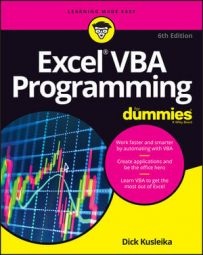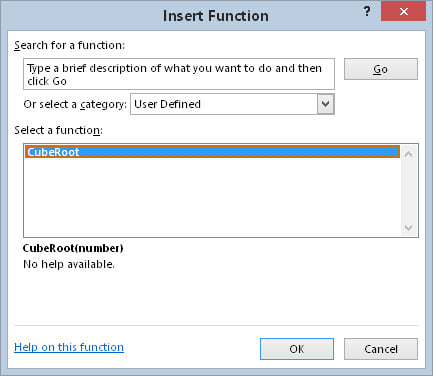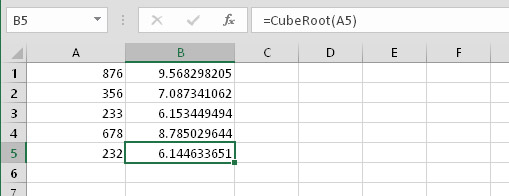##### Excel VBA Programming For DummiesIn VBA programming, a function returns a value. You can execute Function procedures and call the function in Excel 2016. Functions, unlike Sub procedures, can be executed in only two ways:
• By calling the function from another Sub procedure or Function procedure

• By using the function in a worksheet formula

Try this simple function. Enter it in a VBA module:
```Function CubeRoot(number)
CubeRoot = number ^ (1 / 3)
End Function```
This function is pretty wimpy; it merely calculates the cube root of the number passed to it as its argument. It does, however, provide a starting point for understanding functions. It also illustrates an important concept about functions: how to return the value. (You do remember that a function returns a value, right?)

Notice that the single line of code that makes up this Function procedure performs a calculation. The result of the math (number to the power of 1/3) is assigned to the variable CubeRoot. Not coincidentally, CubeRoot is also the name of the function. To tell the function what value to return, you assign that value to the name of the function.

## Calling the function from a Sub procedure

Because you can’t execute a function directly, you must call it from another procedure. Enter the following simple procedure in the same VBA module that contains the CubeRoot function:
```Sub CallerSub()
Ans = CubeRoot(125)
MsgBox Ans
End Sub```
When you execute the CallerSub procedure, Excel displays a message box that contains the value of the Ans variable, which is 5.

Here’s what’s going on: The CubeRoot function is executed, and it receives an argument of 125. The calculation is performed by the function’s code (using the value passed as an argument), and the function’s returned value is assigned to the Ans variable. The MsgBox function then displays the value of the Ans variable.

Try changing the argument that’s passed to the CubeRoot function and run the CallerSub macro again. It works just like it should — assuming that you give the function a valid argument (a positive number).

By the way, the CallerSub procedure could be simplified a bit. The Ans variable is not really required unless your code will use that variable later on. You could use this single statement to obtain the same result:

`  MsgBox CubeRoot(125)`

## Calling a function from a worksheet formula

Now it’s time to call this VBA Function procedure from a worksheet formula. Activate a worksheet in the same workbook that holds the CubeRoot function definition. Then enter the following formula in any cell:
`=CubeRoot(1728)`
The cell displays 12, which is indeed the cube root of 1,728.

As you might expect, you can use a cell reference as the argument for the CubeRoot function. For example, if cell A1 contains a value, you can enter =CubeRoot(A1). In this case, the function returns the number obtained by calculating the cube root of the value in A1.

You can use this function any number of times in the worksheet. Like Excel’s built-in functions, your custom functions appear in the Insert Function dialog box. Click the Insert Function toolbar button, and choose the User Defined category. The Insert Function dialog box lists your very own function.The CubeRoot function appears in the User Defined category of the Insert Function dialog box.
If you want the Insert Function dialog box to display a description of the function, follow these steps:
1. Choose Developer → Code → Macros.

Excel displays the Macro dialog box, but CubeRoot doesn’t appear in the list. (CubeRoot is a Function procedure, and this list shows only Sub procedures.) Don’t fret.

2. Type the word CubeRoot in the Macro Name box.

3. Click the Options button.

4. Enter a description of the function in the Description box.

5. Click OK to close the Macro Options dialog box.

6. Close the Macro dialog box by clicking the Cancel button.

This descriptive text now appears in the Insert Function dialog box.

Check out the CubeRoot function being used in worksheet formulas.Using the CubeRoot function in formulas.